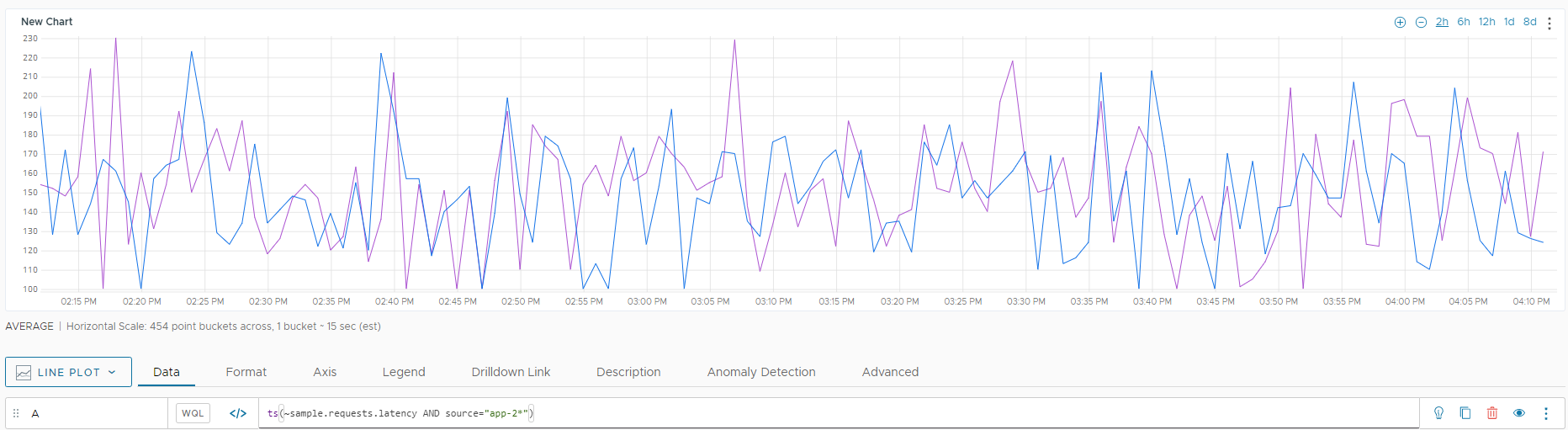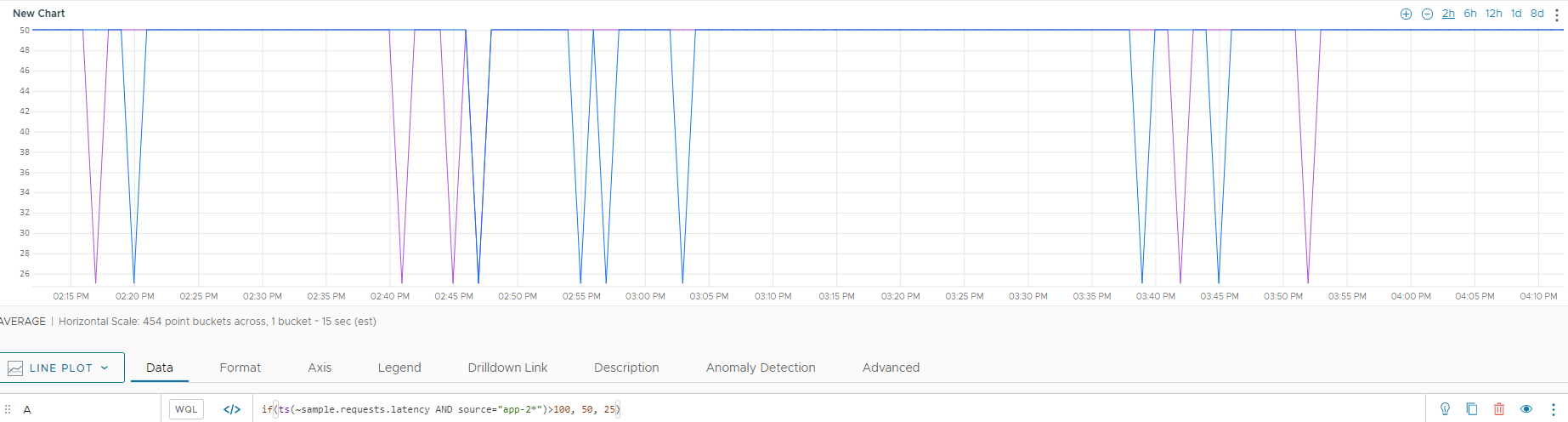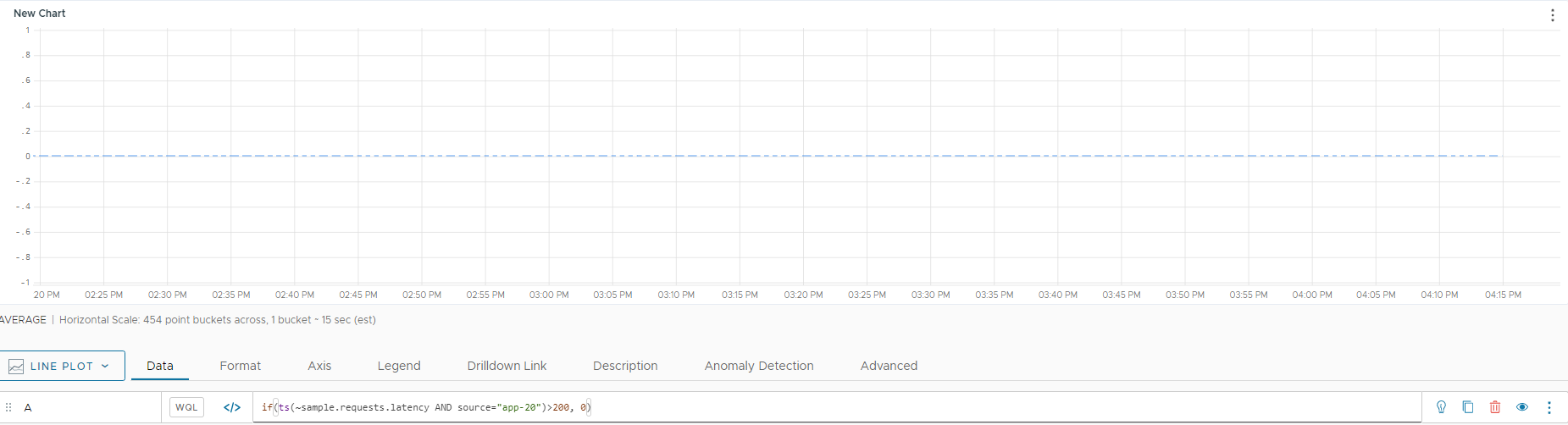Reference to the if() function

## Summary

``````if(<condition-tsExpression>, <then-tsExpression>, [<else-tsExpression>])
``````

Returns the points from `then-tsExpression` while `condition-tsExpression` returns non-zero values. Returns points from `else-tsExpression` otherwise.

## Parameters

ParameterDescription
condition-tsExpression Expression that describes the time series to be used as the condition. For example, if the condition is cpu.loadavg.1m>100, then the condition evaluates to true if the load is greater than 100, and to false otherwise.
This expression typically uses a comparison operator.
then-tsExpression Required expression that describes the time series to return when the condition is met.
• If condition-tsExpression is true for a point, we return the corresponding point from then-tsExpression.
• If condition-tsExpression is false for a point, we return nothing for that point.
else-tsExpression Optional expression that describes the time series to return when the condition is not met.
If you include else-tsExpression:
• If condition-tsExpression is true for a point, we return the corresponding point from then-tsExpression, that is, we ignore else-tsExpression
• If condition-tsExpression is false for a point, we return the corresponding point from else-tsExpression. That means we return return a continuous stream of points that change depending on the value of the condition.

## Description

The `if()` conditional function returns data values based on the specified condition. You can determine what values are returned if the condition is true, and what values are returned if the condition is false. The query engine treats a 0 value as false, and all other values as true.

Each of the tsExpressions can be a constant or a query that returns one or more non-constant time series.

### Interpolation

We use interpolation to connect points in each of the expressions. We use interpolated values in `conditional-tsExpression` to determine whether to return values from `then-tsExpression` or `else-tsExpression`. For example:

• Assume you have an `if()` query like the following:

`if(ts(my.metricA) > 0, ts(my.metricB), ts(my.metricC))`.

• `my.metricA` reports a value of 0 at 12:00pm and `my.metricC` reports values at 11:59am and 12:01pm.
• Because `my.metricA` is false, we show `my.metricC`and we show an interpolated value for `my.metricC` at 12:00pm.

### Series Matching and if()

We perform series matching when 2 or more parameters are `tsExpression`s that each describe multiple time series.

For example, assume we have a query that’s structured like this:

`if(ts(my.metricA) > 100, ts(my.metricB))`

• `my.metricA` describes 100 time series
• `my.metricB` describes 50 time series,
• If some elements in the `my.metricA` time series, for example, the hosts, map to elements in the time series in `myMetricB`, then we can return `myMetricB` time series for those series where `my.metricA` is greater than 100.
• However, if the time series don’t include elements that map, then it’s not possible to determine what to return if the condition is met.

## Examples

The following example set starts with a simple metric, which looks like this:Now we use an `if` condition that returns a value of 50 for any point that’s greater than 100, and a value of 25 otherwise. Here, the behavior of the two time series diverges: For one, the value alternates between 25 and 50. The other time series is always greater than 100 and displays a single orange line.Finally, we look at an example that does not use an `else-tsExpression`. For this case, we’ve limited the query to one time series.